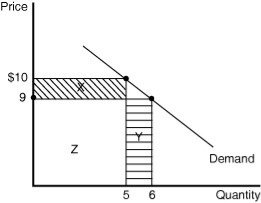• FAQ
• Contact/ Homework Answers / Economics / 31) Which of the following not a characteristic of monopolistic
Not my Question
Flag Content

# Question

31) Which of the following is not a characteristic of monopolistic competition?

A) Firms are price takers.

B) There are many buyers and sellers.

C) Barriers to entry are low.

D) Firms sell similar, but not identical, products.

32) Which of the following is not a characteristic of monopolistic competition?

A) There are many buyers and sellers.

B) There are low barriers to entry.

C) Average revenue is equal to price.

D) The products sold by all firms are identical.

33) In the United States, the average person mostly patronizes firms that operate in

A) perfectly competitive markets.

B) monopolistically competitive markets.

C) oligopoly markets.

D) monopoly markets.

34) Which of the following is the best example of a firm that competes in a monopolistically competitive market?

A) the U.S. Postal Service

B) Microsoft

C) a movie theater

D) an automobile manufacturer

35) In San Francisco there are many restaurants that specialize in a wide variety of cuisines. Patronage at these restaurants is influenced by factors such as tastes, price and location. This market is

A) perfectly competitive.

B) monopolistically competitive.

C) oligopolistic.

D) monopolistic.

36) When a monopolistically competitive firm lowers its price, one good thing happens to the firm. What is this "one good thing" called?

A) the output effect

B) the price effect

C) the income effect

D) the substitution effect

37) When a monopolistically competitive firm lowers it price one bad thing happens to the firm. What is this "one bad thing" called?

A) the output effect

B) the income effect

C) the substitution effect

D) the price effect

Figure 13-338) Refer to Figure 13-3.  The marginal revenue from one additional unit sold is the sum of the gain in revenue from selling the additional unit and the loss in revenue from having to charge a lower price to sell the additional unit. Based on the diagram in the figure,

A) X represents the gain (price effect) and Y the loss (output effect).

B) X + Z represents the loss (output effect) and Y the gain (price effect).

C) Y represents the gain (output effect) and X the loss (price effect).

D) X represents the loss (price effect) and Y + Z the gain (output effect).

39) Refer to Figure 13-3.  What is the marginal revenue of the sixth unit of output?

A) \$4

B) \$5

C) \$9

D) \$54

40) For the monopolistically competitive firm,

A) Price (P) = Marginal Revenue (MR) = Average Revenue (AR).

B) P = MR > AR.

C) P = AR > MR.

D) P > MR = AR.

## Solution 5 (1 Ratings )

Solved
Economics 4 Months Ago 43 Views